# Viscosity

ViscosityA simulation of substances with different viscosities. The substance on the left has lower viscosity than the substance on the right
Common symbols
η, μ
SI unit Pa·s = (N·s)/m2 = kg/(s·m)
Derivations from
other quantities
μ = G·t

The viscosity of a fluid is a measure of its resistance to gradual deformation by shear stress or tensile stress. For liquids, it corresponds to the informal concept of "thickness"; for example, honey has a much higher viscosity than water.

Viscosity is a property of the fluid which opposes the relative motion between the two surfaces of the fluid in a fluid that are moving at different velocities. When the fluid is forced through a tube, the particles which compose the fluid generally move more quickly near the tube's axis and more slowly near its walls; therefore some stress (such as a pressure difference between the two ends of the tube) is needed to overcome the friction between particle layers to keep the fluid moving. For a given velocity pattern, the stress required is proportional to the fluid's viscosity.

A fluid that has no resistance to shear stress is known as an ideal or inviscid fluid. Zero viscosity is observed only at very low temperatures in superfluids. Otherwise, all fluids have positive viscosity, and are technically said to be viscous or viscid. In common parlance, however, a liquid is said to be viscous if its viscosity is substantially greater than that of water, and may be described as mobile if the viscosity is noticeably less than water. A fluid with a relatively high viscosity, such as pitch, may appear to be a solid.

## Etymology

The word "viscosity" is derived from the Latin "viscum", meaning mistletoe and also a viscous glue made from mistletoe berries.

## Definition

### Dynamic (shear) viscosity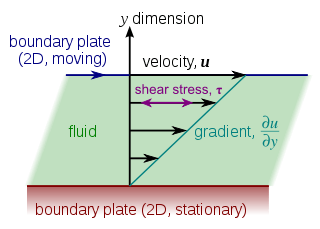Laminar shear of fluid between two plates. Friction between the fluid and the moving boundaries/plates causes the fluid to shear. The force required for this action is a measure of the fluid's viscosity.In a general parallel flow (such as could occur in a straight pipe), the shear stress is proportional to the gradient of the velocity

The dynamic (shear) viscosity of a fluid expresses its resistance to shearing flows, where adjacent layers move parallel to each other with different speeds. It can be defined through the idealized situation known as a Couette flow, where a layer of fluid is trapped between two horizontal plates, one fixed and one moving horizontally at constant speed. This fluid has to be homogeneous in the layer and at different shear stresses. (The plates are assumed to be very large, so that one need not consider what happens near their edges.)

If the speed of the top plate is small enough, the fluid particles will move parallel to it, and their speed will vary linearly from zero at the bottom to u at the top. Each layer of fluid will move faster than the one just below it, and friction between them will give rise to a force resisting their relative motion. In particular, the fluid will apply on the top plate a force in the direction opposite to its motion, and an equal but opposite one to the bottom plate. An external force is therefore required in order to keep the top plate moving at constant speed.

The magnitude F of this force is found to be proportional to the speed u and the area A of each plate, and inversely proportional to their separation y:The proportionality factor μ in this formula is the viscosity (specifically, the dynamic viscosity) of the fluid.

The ratio u/y is called the rate of shear deformation or shear velocity, and is the derivative of the fluid speed in the direction perpendicular to the plates. Isaac Newton expressed the viscous forces by the differential equationwhere τ = F/A, and u/y is the local shear velocity. This formula assumes that the flow is moving along parallel lines and the y-axis, perpendicular to the flow, points in the direction of maximum shear velocity. This equation can be used where the velocity does not vary linearly with y, such as in fluid flowing through a pipe.

Use of the Greek letter mu (μ) for the dynamic stress viscosity is common among mechanical and chemical engineers, as well as physicists. However, the Greek letter eta (η) is also used by chemists, physicists, and the IUPAC.

### Kinematic viscosity

The kinematic viscosity (also called "momentum diffusivity") is the ratio of the dynamic viscosity μ to the density of the fluid ρ. It is usually denoted by the Greek letter nu (ν).It is a convenient concept when analyzing the Reynolds number, which expresses the ratio of the inertial forces to the viscous forces:where L is a typical length scale in the system.

### Bulk viscosity

When a compressible fluid is compressed or expanded evenly, without shear, it may still exhibit a form of internal friction that resists its flow. These forces are related to the rate of compression or expansion by a factor called the volume viscosity, bulk viscosity or second viscosity.

The bulk viscosity is important only when the fluid is being rapidly compressed or expanded, such as in sound and shock waves. Bulk viscosity explains the loss of energy in those waves, as described by Stokes' law of sound attenuation.

### Viscosity tensor

Main article: Viscous stress tensor

In general, the stresses within a flow can be attributed partly to the deformation of the material from some rest state (elastic stress), and partly to the rate of change of the deformation over time (viscous stress). In a fluid, by definition, the elastic stress includes only the hydrostatic pressure.

In very general terms, the fluid's viscosity is the relation between the strain rate and the viscous stress. In the Newtonian fluid model, the relationship is by definition a linear map, described by a viscosity tensor that, multiplied by the strain rate tensor (which is the gradient of the flow's velocity), gives the viscous stress tensor.

The viscosity tensor has nine independent degrees of freedom in general. For isotropic Newtonian fluids, these can be reduced to two independent parameters. The most usual decomposition yields the stress viscosity μ and the bulk viscosity σ.

## Newtonian and non-Newtonian fluids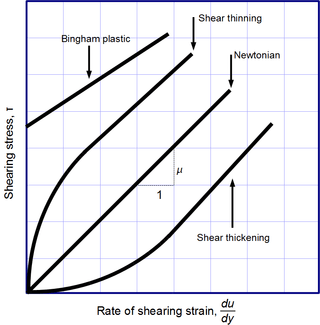Viscosity, the slope of each line, varies among materials

Newton's law of viscosity is a constitutive equation (like Hooke's law, Fick's law, Ohm's law): it is not a fundamental law of nature but an approximation that holds in some materials and fails in others.

A fluid that behaves according to Newton's law, with a viscosity μ that is independent of the stress, is said to be Newtonian. Gases, water, and many common liquids can be considered Newtonian in ordinary conditions and contexts. There are many non-Newtonian fluids that significantly deviate from that law in some way or other. For example:

• Shear-thickening liquids, whose viscosity increases with the rate of shear strain.
• Shear-thinning liquids, whose viscosity decreases with the rate of shear strain.
• Thixotropic liquids, that become less viscous over time when shaken, agitated, or otherwise stressed.
• Rheopectic liquids, that become more viscous over time when shaken, agitated, or otherwise stressed.
• Bingham plastics that behave as a solid at low stresses but flow as a viscous fluid at high stresses.

Shear-thinning liquids are very commonly, but misleadingly, described as thixotropic.

Even for a Newtonian fluid, the viscosity usually depends on its composition and temperature. For gases and other compressible fluids, it depends on temperature and varies very slowly with pressure.

The viscosity of some fluids may depend on other factors. A magnetorheological fluid, for example, becomes thicker when subjected to a magnetic field, possibly to the point of behaving like a solid.

## Viscosity in solids

The viscous forces that arise during fluid flow must not be confused with the elastic forces that arise in a solid in response to shear, compression or extension stresses. While in the latter the stress is proportional to the amount of shear deformation, in a fluid it is proportional to the rate of deformation over time. (For this reason, Maxwell used the term fugitive elasticity for fluid viscosity.)

However, many liquids (including water) will briefly react like elastic solids when subjected to sudden stress. Conversely, many "solids" (even granite) will flow like liquids, albeit very slowly, even under arbitrarily small stress. Such materials are therefore best described as possessing both elasticity (reaction to deformation) and viscosity (reaction to rate of deformation); that is, being viscoelastic.

Indeed, some authors have claimed that amorphous solids, such as glass and many polymers, are actually liquids with a very high viscosity (greater than 1012 Pa·s).  However, other authors dispute this hypothesis, claiming instead that there is some threshold for the stress, below which most solids will not flow at all, and that alleged instances of glass flow in window panes of old buildings are due to the crude manufacturing process of older eras rather than to the viscosity of glass.

Viscoelastic solids may exhibit both shear viscosity and bulk viscosity. The extensional viscosity is a linear combination of the shear and bulk viscosities that describes the reaction of a solid elastic material to elongation. It is widely used for characterizing polymers.

In geology, earth materials that exhibit viscous deformation at least three orders of magnitude greater than their elastic deformation are sometimes called rheids.

## Viscosity measurement

Main article: Viscometer

Viscosity is measured with various types of viscometers and rheometers. A rheometer is used for those fluids that cannot be defined by a single value of viscosity and therefore require more parameters to be set and measured than is the case for a viscometer. Close temperature control of the fluid is essential to acquire accurate measurements, particularly in materials like lubricants, whose viscosity can double with a change of only 5 °C.

For some fluids, viscosity is a constant over a wide range of shear rates (Newtonian fluids). The fluids without a constant viscosity (non-Newtonian fluids) cannot be described by a single number. Non-Newtonian fluids exhibit a variety of different correlations between shear stress and shear rate.

One of the most common instruments for measuring kinematic viscosity is the glass capillary viscometer.

In coating industries, viscosity may be measured with a cup in which the efflux time is measured. There are several sorts of cup – such as the Zahn cup and the Ford viscosity cup – with usage of each type varying mainly according to the industry. The efflux time can also be converted to kinematic viscosities (centistokes, cSt) through the conversion equations.

Also used in coatings, a Stormer viscometer uses load-based rotation in order to determine viscosity. The viscosity is reported in Krebs units (KU), which are unique to Stormer viscometers.

Vibrating viscometers can also be used to measure viscosity. These models such as the Dynatrol use vibration rather than rotation to measure viscosity.

Extensional viscosity can be measured with various rheometers that apply extensional stress.

Volume viscosity can be measured with an acoustic rheometer.

Apparent viscosity is a calculation derived from tests performed on drilling fluid used in oil or gas well development. These calculations and tests help engineers develop and maintain the properties of the drilling fluid to the specifications required.

## Units

### Dynamic viscosity μ

Both the physical unit of dynamic viscosity in SI units, the poiseuille (Pl), and cgs units, the poise (P), are named after Jean Léonard Marie Poiseuille. The poiseuille, which is rarely used, is equivalent to the pascal second (Pa·s), or (N·s)/m2, or kg/(m·s). If a fluid is placed between two plates with distance one meter, and one plate is pushed sideways with a shear stress of one pascal, and it moves at x meters per second, then it has viscosity of 1/x pascal seconds. For example, water at 20 °C has a viscosity of 1.002 mPa·s, while a typical motor oil could have a viscosity of about 250 mPa·s. The units used in practice are either Pa·s and its submultiples or the cgs poise referred to below, and its submultiples.

The cgs physical unit for dynamic viscosity, the poise (P), is also named after Jean Poiseuille. It is more commonly expressed, particularly in ASTM standards, as centipoise (cP) since the latter is equal to the SI multiple millipascal seconds (mPa·s). For example, water at 20 °C has a viscosity of 1.002 mPa·s = 1.0020*nbsp;cP.

1 Pl = 1 Pa·s
1 P = 0.1 Pa·s= 0.1 kg·m−1·s−1
1 cP = 1 mPa·s = 0.001 Pa·s = 0.001 N·s·m−2 = 0.001 kg·m−1·s−1.

### Kinematic viscosity ν

The SI unit of kinematic viscosity is m2/s.

The cgs physical unit for kinematic viscosity is the stokes (St), named after George Gabriel Stokes. It is sometimes expressed in terms of centistokes (cSt). In U.S. usage, stoke is sometimes used as the singular form.

1 St = 1 cm2·s−1 = 10−4 m2·s−1.
1 cSt = 1 mm2·s−1 = 10−6 m2·s−1.

Water at 20 °C has a kinematic viscosity of about 10−6 m2·s−1 or 1 cSt.

The kinematic viscosity is sometimes referred to as diffusivity of momentum, because it is analogous to diffusivity of heat and diffusivity of mass. It is therefore used in dimensionless numbers which compare the ratio of the diffusivities.

### Fluidity

The reciprocal of viscosity is fluidity, usually symbolized by φ = 1/μ or F = 1/μ, depending on the convention used, measured in reciprocal poise (P−1, or cm·s·g−1), sometimes called the rhe. Fluidity is seldom used in engineering practice.

The concept of fluidity can be used to determine the viscosity of an ideal solution. For two components A and B, the fluidity when A and B are mixed iswhich is only slightly simpler than the equivalent equation in terms of viscosity:where χA and χB are the mole fractions of components A and B respectively, and μA and μB are the components' pure viscosities.

### Non-standard units

The Reyn is a British unit of dynamic viscosity.

Viscosity index is a measure for the change of kinematic viscosity with temperature. It is used to characterise lubricating oil in the automotive industry.

At one time the petroleum industry relied on measuring kinematic viscosity by means of the Saybolt viscometer, and expressing kinematic viscosity in units of Saybolt Universal Seconds (SUS). Other abbreviations such as SSU (Saybolt Seconds Universal) or SUV (Saybolt Universal Viscosity) are sometimes used. Kinematic viscosity in centistoke can be converted from SUS according to the arithmetic and the reference table provided in ASTM D 2161.

## Molecular origins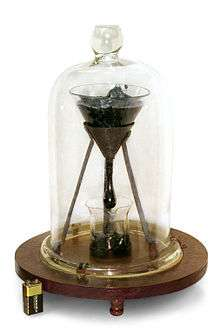Pitch has a viscosity approximately 230 billion (2.3×1011) times that of water.

The viscosity of a system is determined by how molecules constituting the system interact. There are no simple but correct expressions for the viscosity of a fluid. The simplest exact expressions are the Green–Kubo relations for the linear shear viscosity or the Transient Time Correlation Function expressions derived by Evans and Morriss in 1985. Although these expressions are each exact, in order to calculate the viscosity of a dense fluid using these relations currently requires the use of molecular dynamics computer simulations.

### Gases

Viscosity in gases arises principally from the molecular diffusion that transports momentum between layers of flow. The kinetic theory of gases allows accurate prediction of the behavior of gaseous viscosity.

Within the regime where the theory is applicable:

• Viscosity is independent of pressure and
• Viscosity increases as temperature increases.

James Clerk Maxwell published a famous paper in 1866 using the kinetic theory of gases to study gaseous viscosity. To understand why the viscosity is independent of pressure, consider two adjacent boundary layers (A and B) moving with respect to each other. The internal friction (the viscosity) of the gas is determined by the probability a particle of layer A enters layer B with a corresponding transfer of momentum. Maxwell's calculations show that the viscosity coefficient is proportional to the density, the mean free path, and the mean velocity of the atoms. On the other hand, the mean free path is inversely proportional to the density. So an increase in density due to an increase in pressure doesn't result in any change in viscosity.

#### Relation to mean free path of diffusing particles

In relation to diffusion, the kinematic viscosity provides a better understanding of the behavior of mass transport of a dilute species. Viscosity is related to shear stress and the rate of shear in a fluid, which illustrates its dependence on the mean free path, λ, of the diffusing particles.

From fluid mechanics, for a Newtonian fluid, the shear stress, τ, on a unit area moving parallel to itself, is found to be proportional to the rate of change of velocity with distance perpendicular to the unit area:for a unit area parallel to the xz-plane, moving along the x axis. We will derive this formula and show how μ is related to λ.

Interpreting shear stress as the time rate of change of momentum, p, per unit area A (rate of momentum flux) of an arbitrary control surface giveswhere ux is the average velocity, along the x-axis, of fluid molecules hitting the unit area, with respect to the unit area and A is the rate of fluid mass hitting the surface.

By making simplified assumption that the velocity of the molecules depends linearly on the distance they are coming from, the mean velocity depends linearly on the mean distance:.

Further manipulation will show,where

ρ is the density of the fluid,
ū is the root mean square molecular speed: ū = u2,
μ is the dynamic viscosity.

Note, that the mean free path itself typically depends (inversely) on the density.

#### Effect of temperature on the viscosity of a gas

Sutherland's formula can be used to derive the dynamic viscosity of an ideal gas as a function of the temperature:This in turn is equal towhereis a constant for the gas.

in Sutherland's formula:

• μ = dynamic viscosity (Pa·s or μPa·s) at input temperature T,
• μ0 = reference viscosity (in the same units as μ) at reference temperature T0,
• T = input temperature (K),
• T0 = reference temperature (K),
• C = Sutherland's constant for the gaseous material in question.

Valid for temperatures between 0 < T < 555 K with an error due to pressure less than 10% below 3.45 MPa.

According to Sutherland's formula, if the absolute temperature is less than C, the relative change in viscosity for a small change in temperature is greater than the relative change in the absolute temperature, but it is smaller when T is above C. The kinematic viscosity though always increases faster than the temperature (that is, d log(ν)/d log(T) is greater than 1).

Sutherland's constant, reference values and λ values for some gases:

Gas C (K) T0 (K) μ0 (μPa·s) λ (μPa·s·K12)
air 120 291.15 18.27 1.512041288
nitrogen 111 300.55 17.81 1.406732195
oxygen 127 292.25 20.18 1.693411300
carbon dioxide 240 293.15 14.8 1.572085931
carbon monoxide 118 288.15 17.2 1.428193225
hydrogen 72 293.85 8.76 0.636236562
ammonia 370 293.15 9.82 1.297443379
sulfur dioxide 416 293.65 12.54 1.768466086
helium 79.4 273 19 1.484381490

#### Viscosity of a dilute gas

The Chapman–Enskog equation may be used to estimate viscosity for a dilute gas. This equation is based on a semi-theoretical assumption by Chapman and Enskog. The equation requires three empirically determined parameters: the collision diameter (σ), the maximum energy of attraction divided by the Boltzmann constant (ε/к) and the collision integral (ω(T*)).with

• T* = κT/ε is reduced temperature (dimensionless),
• μ0 is viscosity for dilute gas (μPa·s),
• M is molecular mass (g/mol),
• T is temperature (K),
• σ is the collision diameter (Å),
• ε/к is the maximum energy of attraction divided by the Boltzmann constant (K),
• ωμ is the collision integral.

### Liquids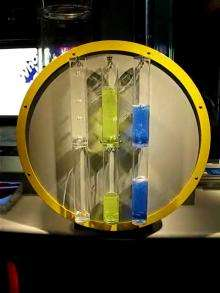Video showing three liquids with different viscosities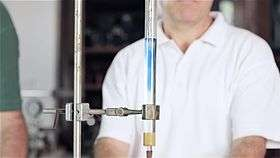Experiment showing the behaviour of a viscous fluid with blue dye for visibility. Performed by Prof. Oliver Zajkov at the Physics Institute at the Ss. Cyril and Methodius University of Skopje, Macedonia.

In liquids, the additional forces between molecules become important. This leads to an additional contribution to the shear stress though the exact mechanics of this are still controversial. Thus, in liquids:

• Viscosity is independent of pressure (except at very high pressure); and
• Viscosity tends to fall as temperature increases (for example, water viscosity goes from 1.79 cP to 0.28 cP in the temperature range from 0 °C to 100 °C); see temperature dependence of liquid viscosity for more details.

The dynamic viscosities of liquids are typically several orders of magnitude higher than dynamic viscosities of gases.

#### Viscosity of blends of liquids

The viscosity of the blend of two or more liquids can be estimated using the Refutas equation. The calculation is carried out in three steps.

The first step is to calculate the viscosity blending number (VBN) (also called the viscosity blending index) of each component of the blend:(1)

where ν is the kinematic viscosity in centistokes (cSt). It is important that the kinematic viscosity of each component of the blend be obtained at the same temperature.

The next step is to calculate the VBN of the blend, using this equation:(2)

where xX is the mass fraction of each component of the blend.

Once the viscosity blending number of a blend has been calculated using equation (2), the final step is to determine the kinematic viscosity of the blend by solving equation (1) for ν:(3)

where VBNBlend is the viscosity blending number of the blend.

## Viscosity of selected substances

### Air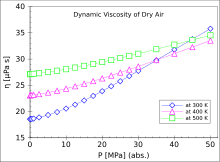Pressure dependence of the dynamic viscosity of dry air at the temperatures of 300, 400 and 500 kelvins

The viscosity of air depends mostly on the temperature. At 15 °C, the viscosity of air is 1.81×10−5 kg/(m·s), 18.1 μPa·s or 1.81×10−5 Pa·s. The kinematic viscosity at 15 °C is 1.48×10−5 m2/s or 14.8 cSt. At 25 °C, the viscosity is 18.6 μPa·s and the kinematic viscosity 15.7 cSt.

### Water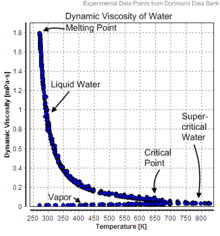Dynamic viscosity of water

The dynamic viscosity of water is 8.90×10−4 Pa·s or 8.90×10−3 dyn·s/cm2 or 0.890 cP at about 25 °C.

As a function of temperature T (in kelvins): μ = A × 10B/(TC), where A = 2.414×10−5 Pa·s, B = 247.8 K, and C = 140 K.

Viscosity of liquid water at different temperatures up to the normal boiling point is listed below.

Viscosity of water at various temperatures
Temperature (°C) Viscosity (mPa·s)
10 1.308
20 1.002
30 0.7978
40 0.6531
50 0.5471
60 0.4658
70 0.4044
80 0.3550
90 0.3150
100 0.2822

### Other substances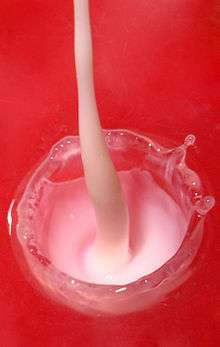Example of the viscosity of milk and water. Liquids with higher viscosities make smaller splashes when poured at the same velocity.Honey being drizzled.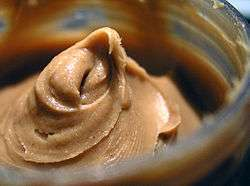Peanut butter is a semi-solid and can therefore hold peaks.

Some dynamic viscosities of Newtonian fluids are listed below:

Viscosity of selected gases at 100 kPa, (μPa·s)
Gas at 0 °C (273 K) at 27 °C (300 K)
air 17.4 18.6
hydrogen 8.4 9.0
helium 20.0
argon 22.9
xenon 21.2 23.2
carbon dioxide 15.0
methane 11.2
ethane 9.5
Viscosity of fluids with variable compositions
Fluid Viscosity (Pa·s) Viscosity (cP)
blood (37 °C) 3×10−34×10−3 3–4
honey 2–10 2000–10000
molasses 5–10 5000–10000
molten glass 10–1000 100001000000
chocolate syrup 10–25 1000025000
molten chocolate[lower-alpha 1] 45–130 45000130000
ketchup[lower-alpha 1] 50–100 50000100000
lard ≈ 100 100000
peanut butter[lower-alpha 1] ≈ 250 250000
shortening[lower-alpha 1] ≈ 250 250000
Viscosity of liquids
(at 25 °C unless otherwise specified)
Liquid Viscosity (Pa·s) Viscosity (cP)
acetone 3.06×10−4 0.306
benzene 6.04×10−4 0.604
castor oil 0.985 985
corn syrup 1.3806 1380.6
ethanol 1.074×10−3 1.074
ethylene glycol 1.61×10−2 16.1
glycerol (at 20 °C) 1.2 1200
HFO-380 2.022 2022
mercury 1.526×10−3 1.526
methanol 5.44×10−4 0.544
motor oil SAE 10 (20 °C) 0.065 65
motor oil SAE 40 (20 °C) 0.319 319
nitrobenzene 1.863×10−3 1.863
liquid nitrogen (−196 °C) 1.58×10−4 0.158
propanol 1.945×10−3 1.945
olive oil 0.081 81
pitch 2.3×108 2.30×1011
sulfuric acid 2.42×10−2 24.2
water 8.94×10−4 0.894
Viscosity of solids
Solid Viscosity (Pa·s) Temperature (°C)
asthenosphere 7.0×1019 900
upper mantle 7×10201×1021 1300–3000
lower mantle 1×10212×1021 3000–4000
1. These materials are highly non-Newtonian.

## Viscosity of slurry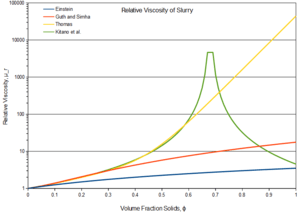Plot of slurry relative viscosity μr as calculated by empirical correlations from Einstein, Guth and Simha, Thomas, and Kitano et al..

The term slurry describes mixtures of a liquid and solid particles that retain some fluidity. The viscosity of slurry can be described as relative to the viscosity of the liquid phase:where μs and μl are respectively the dynamic viscosity of the slurry and liquid (Pa·s), and μr is the relative viscosity (dimensionless).

Depending on the size and concentration of the solid particles, several models exist that describe the relative viscosity as a function of volume fraction φ of solid particles.

In the case of extremely low concentrations of fine particles, Einstein's equation may be used:In the case of higher concentrations, a modified equation was proposed by Guth and Simha, which takes into account interaction between the solid particles:Further modification of this equation was proposed by Thomas from the fitting of empirical data:where A = 0.00273 and B = 16.6.

In the case of high shear stress (above 1 kPa), another empirical equation was proposed by Kitano et al. for polymer melts:where A = 0.68 for smooth spherical particles.

## Viscosity of amorphous materials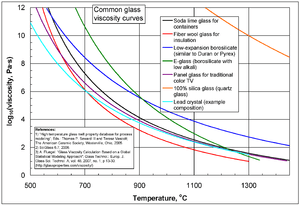Common glass viscosity curves.

Viscous flow in amorphous materials (e.g. in glasses and melts) is a thermally activated process:where Q is activation energy, T is temperature, R is the molar gas constant and A is approximately a constant.

The viscous flow in amorphous materials is characterized by a deviation from the Arrhenius-type behavior: Q changes from a high value QH at low temperatures (in the glassy state) to a low value QL at high temperatures (in the liquid state). Depending on this change, amorphous materials are classified as either

• strong when: QHQL < QL or
• fragile when: QHQLQL.

The fragility of amorphous materials is numerically characterized by Doremus’ fragility ratio:and strong materials have RD < 2 whereas fragile materials have RD ≥ 2.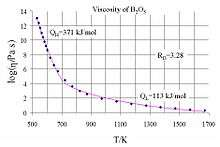Common logarithm of viscosity against temperature for B2O3, showing two regimes

The viscosity of amorphous materials is quite exactly described by a two-exponential equation:with constants A1, A2, B, C and D related to thermodynamic parameters of joining bonds of an amorphous material.

Not very far from the glass transition temperature, Tg, this equation can be approximated by a Vogel–Fulcher–Tammann (VFT) equation.

If the temperature is significantly lower than the glass transition temperature, TTg, then the two-exponential equation simplifies to an Arrhenius-type equation:withwhere Hd is the enthalpy of formation of broken bonds (termed configurons) and Hm is the enthalpy of their motion.

When the temperature is less than the glass transition temperature, T < Tg, the activation energy of viscosity is high because the amorphous materials are in the glassy state and most of their joining bonds are intact.

If the temperature is much higher than the glass transition temperature, TTg, the two-exponential equation also simplifies to an Arrhenius-type equation:withWhen the temperature is higher than the glass transition temperature, T > Tg, the activation energy of viscosity is low because amorphous materials are melted and have most of their joining bonds broken, which facilitates flow.

## Eddy viscosity

In the study of turbulence in fluids, a common practical strategy for calculation is to ignore the small-scale vortices (or eddies) in the motion and to calculate a large-scale motion with an eddy viscosity that characterizes the transport and dissipation of energy in the smaller-scale flow (see large eddy simulation). Values of eddy viscosity used in modeling ocean circulation may be from 5×104 to 1×106 Pa·s depending upon the resolution of the numerical grid.

## References

1. "viscosity". Merriam-Webster Dictionary.
2. Symon, Keith (1971). Mechanics (3rd ed.). Addison-Wesley. ISBN 0-201-07392-7.
3. "viscous". Etymonline.com. Retrieved 2010-09-14.
4. Streeter, Victor Lyle; Wylie, E. Benjamin; Bedford, Keith W. (1998). Fluid Mechanics. McGraw-Hill. ISBN 0-07-062537-9.
5. Holman, J. P. (2002). Heat Transfer. McGraw-Hill. ISBN 0-07-122621-4.
6. Incropera, Frank P.; DeWitt, David P. (2007). Fundamentals of Heat and Mass Transfer. Wiley. ISBN 0-471-45728-0.
7. Nič, Miloslav; Jirát, Jiří; Košata, Bedřich; Jenkins, Aubrey, eds. (1997). "dynamic viscosity, η". IUPAC Compendium of Chemical Terminology. Oxford: Blackwell Scientific Publications. doi:10.1351/goldbook. ISBN 0-9678550-9-8.
8. Kumagai, Naoichi; Sasajima, Sadao; Ito, Hidebumi (15 February 1978). "Long-term Creep of Rocks: Results with Large Specimens Obtained in about 20 Years and Those with Small Specimens in about 3 Years". Journal of the Society of Materials Science (Japan). Japan Energy Society. 27 (293): 157–161. Retrieved 2008-06-16.
9. Elert, Glenn. "Viscosity". The Physics Hypertextbook.
10. Gibbs, Philip. "Is Glass a Liquid or a Solid?". Retrieved 2007-07-31.
11. Plumb, Robert C. (1989). "Antique windowpanes and the flow of supercooled liquids". Journal of Chemical Education. 66 (12): 994. Bibcode:1989JChEd..66..994P. doi:10.1021/ed066p994.
12. Scherer, George W.; Pardenek, Sandra A.; Swiatek, Rose M. (1988). "Viscoelasticity in silica gel". Journal of Non-Crystalline Solids. 107: 14. Bibcode:1988JNCS..107...14S. doi:10.1016/0022-3093(88)90086-5.
13. Grossi, Marco; Riccò, Bruno (2016). "A portable electronic system for in-situ measurements of oil concentration in MetalWorking fluids". Sensors and Actuators A: Physical. 243: 7–14. doi:10.1016/j.sna.2016.03.006.
14. "Viscosity" (PDF). BYK-Gardner.
15. Serway, Raymond A. (1996). Physics for Scientists & Engineers (4th ed.). Saunders College Publishing. ISBN 0-03-005932-1.
16. "IUPAC definition of the Poise". Retrieved 2010-09-14.
17. ASTM D 2161 (2005) "Standard Practice for Conversion of Kinematic Viscosity to Saybolt Universal Viscosity or to Saybolt Furol Viscosity", p. 1
18. "Quantities and Units of Viscosity". Uniteasy.com. Retrieved 2010-09-14.
19. Edgeworth, R.; Dalton, B. J.; Parnell, T. (1984). "The pitch drop experiment". European Journal of Physics. 1984: 198–200. Retrieved 2009-03-31.
20. Evans, Denis J.; Morriss, Gary P. (October 15, 1988). "Transient-time-correlation functions and the rheology of fluids". Physical Review A. 38 (8): 4142–4148. Bibcode:1988PhRvA..38.4142E. doi:10.1103/PhysRevA.38.4142. PMID 9900865. Retrieved 2012-10-24.
21. Elert, Glenn. "The Physics Hypertextbook – Viscosity". Physics.info. Retrieved 2010-09-14.
22. Maxwell, J. C. (1866). "On the viscosity or internal friction of air and other gases". Philosophical Transactions of the Royal Society of London. 156: 249–268. doi:10.1098/rstl.1866.0013.
23. Salmon, R. L. (1998). Lectures on geophysical fluid dynamics. Oxford University Press. p. 23–26. ISBN 0-19-510808-6.
24. Smits, Alexander J.; Dussauge, Jean-Paul (2006). Turbulent shear layers in supersonic flow. Birkhäuser. p. 46. ISBN 0-387-26140-0.
25. Kim, Youn J.; Kim, You-Jae; Han, J.-G. (1970). "Numerical analysis of flow characteristics of an atmospheric plasma torch". 12th International Congress on Plasma Physics, 25–29 October 2004, Nice (France). arXiv:physics/0410237. Bibcode:2004physics..10237K.
26. "Viscosity of liquids and gases". GSU Hyperphysics.
27. Hirshfelder, J. O.; Curtis, C. F.; Bird, R. B. (1964). Molecular theory of gases and liquids (1st ed.). Wiley. ISBN 0-471-40065-3.
28. Maples, Robert E. (2000). Petroleum Refinery Process Economics (2nd ed.). Pennwell Books. ISBN 0-87814-779-9.
29. Lide, D. R., ed. (2005). CRC Handbook of Chemistry and Physics (86th ed.). Boca Raton (FL): CRC Press. ISBN 0-8493-0486-5.
30. Yanniotis, S.; Skaltsi, S.; Karaburnioti, S. (February 2006). "Effect of moisture content on the viscosity of honey at different temperatures". Journal of Food Engineering. 72 (4): 372–377. doi:10.1016/j.jfoodeng.2004.12.017.
31. "Chocolate Processing". Brookfield Engineering website. Retrieved 2007-12-03.
32. Fjeldskaar, W. (1994). "Viscosity and thickness of the asthenosphere detected from the Fennoscandian uplift". Earth and Planetary Science Letters. 126 (4): 399–410. Bibcode:1994E&PSL.126..399F. doi:10.1016/0012-821X(94)90120-1.
33. Einstein, A. (1906). "Eine neue Bestimmung der Moleküldimensionen". Annalen der Physik. 19 (2): 289. Bibcode:1906AnP...324..289E. doi:10.1002/andp.19063240204.
34. Guth, E.; Simha, R. (1936). "Untersuchungen über die Viskosität von Suspensionen und Lösungen. 3. Über die Viskosität von Kugelsuspensionen". Kolloid Z. 74 (3): 266. doi:10.1007/BF01428643.
35. Thomas, D. G. (1965). "Transport characteristics of suspension: VIII. A note on the viscosity of Newtonian suspensions of uniform spherical particles". J. Colloid Sci. 20 (3): 267. doi:10.1016/0095-8522(65)90016-4.
36. Kitano, T.; Kataoka, T.; Shirota, T. (1981). "An empirical equation of the relative viscosity of polymer melts filled with various inorganic fillers". Rheologica Acta. 20 (2): 207. doi:10.1007/BF01513064.
37. Fluegel, Alexander. "Viscosity calculation of glasses". Glassproperties.com. Retrieved 2010-09-14.
38. Doremus, R. H. (2002). "Viscosity of silica". J. Appl. Phys. 92 (12): 7619–7629. Bibcode:2002JAP....92.7619D. doi:10.1063/1.1515132.
39. Ojovan, M. I.; Lee, W. E. (2004). "Viscosity of network liquids within Doremus approach". J. Appl. Phys. 95 (7): 3803–3810. Bibcode:2004JAP....95.3803O. doi:10.1063/1.1647260.
40. Ojovan, M. I.; Travis, K. P.; Hand, R. J. (2000). "Thermodynamic parameters of bonds in glassy materials from viscosity-temperature relationships". J. Phys.: Condensed Matter. 19 (41): 415107. Bibcode:2007JPCM...19O5107O. doi:10.1088/0953-8984/19/41/415107.
41. "Configuron". Wikidoc.Look up viscosity in Wiktionary, the free dictionary.Wikisource has the text of The New Student's Reference Work article Viscosity of Liquids.